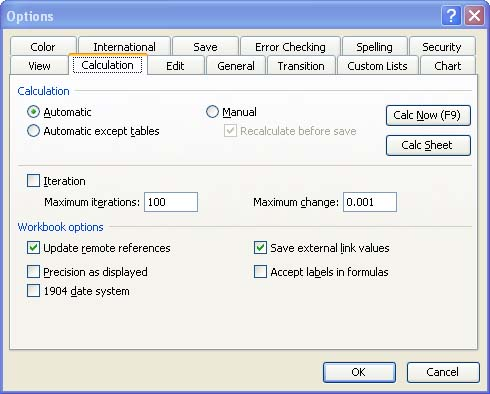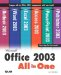# Recalculating the Worksheet

Excel automatically recalculates the results in your worksheet every time you enter a new value or edit a value or formula. This is fine for most workbooks. However, if you have a computer with limited memory and processing power, you might find that having Excel recalculate all the results in a very large worksheet every time you make a change means that you are sitting and waiting for Excel to complete the recalculation.

You can turn off the automatic recalculation. However, this won't be necessary except in situations where you are working with huge workbooks that contain a very large number of formulas, functions, and data. Turning off the automatic calculation feature also means that you must remember to manually recalculate the values in the worksheet before you print. To change the recalculation setting, take the following steps:

1. Open the Tools menu and choose Options .

2. Click the Calculation tab to display the options shown in Figure 4.3.

##### Figure 4.3. You can turn off the automatic recalculation feature.3. Select one of the following Calculation options:

• Automatic This is the default setting. It recalculates the entire workbook each time you edit or enter a formula.

• Automatic Except Tables This automatically recalculates everything except formulas in a data table (data tables are used to provide a range of data for one formula and function and are used for an advanced Excel feature called "What If Analysis").

• Manual This option tells Excel to recalculate only when you say so. To recalculate manually, press the F9 key. When this option is selected, you can turn the Recalculate Before Save option off or on.

4. Click OK.Microsoft Office 2003 All-in-One
ISBN: B005HKSHB2
EAN: N/A
Year: 2002
Pages: 660
Authors: Joe Habraken

Similar book on Amazon